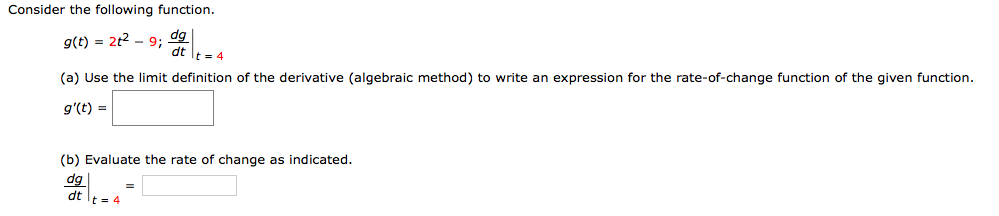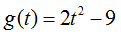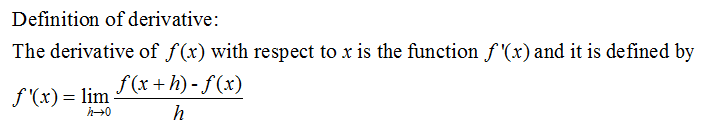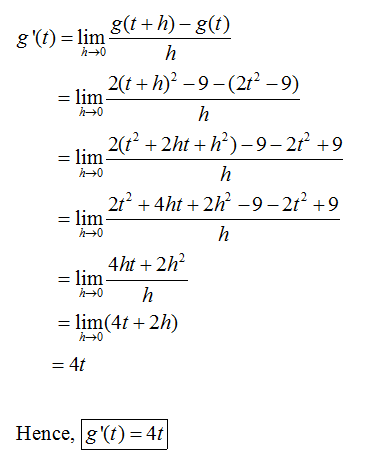# Consider the following function.g(t) = 2t2 - 9;dp.dtIt = 4(a) Use the limit definition of the derivative (algebraic method) to write an expression for the rate-of-change function of the given function.g'(t) =(b) Evaluate the rate of change as indicated.dgdt t= 4

Question
1 viewshelp_outlineImage TranscriptioncloseConsider the following function. g(t) = 2t2 - 9; dp. dt It = 4 (a) Use the limit definition of the derivative (algebraic method) to write an expression for the rate-of-change function of the given function. g'(t) = (b) Evaluate the rate of change as indicated. dg dt t= 4 fullscreen
check_circle

Step 1

Given: the functionStep 2

Concept used:Step 3

The rate change of function:...

### Want to see the full answer?

See Solution

#### Want to see this answer and more?

Solutions are written by subject experts who are available 24/7. Questions are typically answered within 1 hour.*

See Solution
*Response times may vary by subject and question.
Tagged in